AP State Syllabus AP Board 9th Class Physical Science Solutions Chapter 11 Sound Textbook Questions and Answers.

## AP State Syllabus 9th Class Physical Science Solutions 11th Lesson Sound

### 9th Class Physical Science 11th Lesson Sound Textbook Questions and Answers

Pick out the correct answer :

Question 1.
When we say sound travels in a medium (AS 1)
A) the medium travels
B) the particles of the medium travel
C) the source travels
D) the disturbance travels
D) the disturbance travels

Question 2.
A sound wave consists of (AS 1)
A) number of compression pulses only
B) number of rarefaction pulses only
C) number of compression and rarefaction pulses one after the other
D) vacuum only
C) number of compression and rarefaction pulses one after the otherQuestion 3.
Hertz stands for oscillations per (AS 1)
A) second
B) minute
C) hour
D) milli second
A)second

Question 4.
When we increase the loudness of sound of a TV, the property of sound that changes is (AS 1)
A) amplitude
B) frequency
C) wavelength
D) speed
A) amplitude

Question 5.
The characteristic of the sound that describes how the brain interprets the frequency of sound is called (AS 1)
A) pitch
B) loudness
C) quality
D) sound
A) pitchQuestion 6.
In a stethoscope, sound of heart beats travel through stethoscope tube (AS 1)
A) by bending along the tube
B) in a straight line
C) undergoing multiple reflections
D) all of the above
C) undergoing multiple reflections

Question 7.
Explain the following terms : (AS 1)
a) amplitude
b) wavelength
c) frequency
a) Amplitude :
The maximum variation in density or pressure from the mean value is called amplitude.
(or)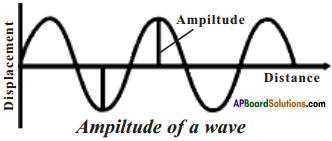The maximum disturbance of particles of a medium from their mean position is called amplitude.

b) Wavelength :
The distance between two consecutive compressions or two consecutive rarefactions is called the wavelength of a sound wave, denoted by W. Wavelength is measured in ‘meters’.

c) Frequency :

1. The number of oscillations of the density of the medium at a place per unit time is called the frequency of the sound wave.
2. Frequency is denoted by ‘o’.
3. The S.I. unit of frequency is ‘Hertz’.Question 8.
Deduce the relation between wavelength, frequency, and speed of sound. (AS 1)
1) Speed of sound can be defined as the distance by which a point on the wave, such as a compression or rarefaction, travels in unit time.

2) Let the distance travelled by a wave in T seconds = X metres

3) The distance travelled by a wave in 1 second = $$\frac{\lambda}{\mathrm{T}}$$ meters

4) Thus by definition of speed of wave, v = $$\frac{\lambda}{\mathrm{T}}$$ ……….. (1)

5) We know that frequency and time period are related as υ = $$\frac{1}{T}$$ ……….. (2)

6) From (1) and (2) we get v = λ .υ
∴ Speed of sound = Frequency × Wavelength

Question 9.
How are multiple reflections of sound helpful to doctors and engipeeps? (AS 7)
1) Doctors use multiple reflections of sound to hear the sounds produced with in the body using stethoscope.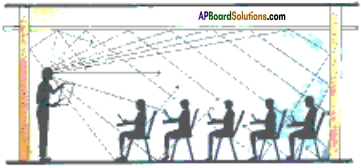2) Doctors can see the images of patient’s organs like liver, gall bladder, uterus, etc. to know the abnormalities in their functioning, using ultrasounds.

3) Engineers use the reflections of sound in designing concert halls and cinema halls.

4) Generally the ceilings of concert halls, conference halls, cinema halls are designed such that sound after reflection reaches all corners of the hall as shown in the figure.Question 10.
Name two quantities that vary periodically at a place in air as a sound wave travels through it. (AS 1)
The two quantities that vary periodically at a place in air as a sound wave travels through it are density and pressure of particles.

Question 11.
Which has larger frequency – infrasonic sound or ultrasonic sound? (AS 7, AS 2)

• Infrasonics are the sounds of frequency less than 20 Hz.
• Ultrasonics are the sounds of frequency greater than 20 kHz.
• Hence the ultrasonics have larger frequency.

Question 12.
The grandparents and parents of two-year-old girl are playing with her in a room. A sound source produces a 28 kHz sound. Who in the room is most likely to hear the sound? (AS 2, AS 7)

• The two-year-old girl is able to hear the sound.
• Children can hear sounds of somewhat higher frequencies up to 30 kHz.

Question 13.
Does the sound follow same laws of reflection as light does? (AS 1)

• Reflection of sound follows the same laws as the reflection of light when sound is reflected.
• The directions in which the sound is incident and reflected make equal angles with the normal to the reflecting surface.Question 14.
Why is soft furnishing avoided in concert halls? (AS 7)

• Sound reflects like the reflection of light.
• But unlike to light, sound reflects more on rough surfaces than soft surfaces.
• In concert halls, sound must undergo multiple reflections, so as to reach all corners of the hall.
• Hence for better reflection, soft furnishing is avoided in concert halls.

Question 15.
Two sources A and B vibrate with the same amplitude. They produce sounds of frequencies 1 kHz and 30 kHz respectively. Which of the two waves will have larger power? (AS 1)
Frequency of source A = 1 kHz; Frequency of source B = 30 kHz

As the speed of wave increases with frequency and both the waves have same amplitude, the sound produced from source B has larger power.Question 16.
What do you understand by a sound wave? (AS 1)

• Sound is produced from a vibrating body.
• It travels through air in the form of a wave.
• Sound waves are longitudinal.

Question 17.
Define the wavelength of a sound wave. How is it related to the frequency and the wave speed? (AS 1)
Wavelength :
The distance between two consecutive compressions or rarefactions is called wavelength.

Relation between wavelength, frequency, and wave speed :

1. Speed of sound can be defined as the distance by which a point on the wave, such as a compression or rarefaction, travels in unit time.
2. Let the distance travelled by a wave in T seconds = λ metres
3. The distance travelled by a wave in 1 second = $$\frac{\lambda}{\mathrm{T}}$$ meters
4. Thus by definition of speed of wave, v = $$\frac{\lambda}{\mathrm{T}}$$ ………… (1)
5. We know that frequency and time period are related as o = $$\frac{1}{T}$$ ……….. (2)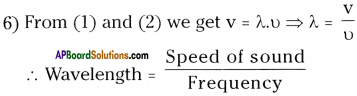Question 18.
Explain how echoes are used by bats to judge the distance of an obstacle in front of them. (AS 1)

• Bats search out prey and fly in dark night by emitting and detecting reflections of ultrasonic waves.
• The high pitched ultrasonic squeaks of the bat are reflected from the obstacles or prey and returned to bat’s ear.
• The nature of reflections tells the bat where the obstacle or prey is and what it is like.
• The bats use ultrasound for navigation and location of the food in dark.Question 19.
With the help of a diagram describe how compression and rarefaction pulses are produced in air near a source of sound. (AS 5)

• Consider a vibrating membrane of a musical instrument like a drum or tabla.
• As it moves back and forth, it produces a sound.
• The figure shows the membrane at different instants and the condition of the air near it at those instants.
• As the membrane moves forward, it pushes the particles of air in the layer in front of it.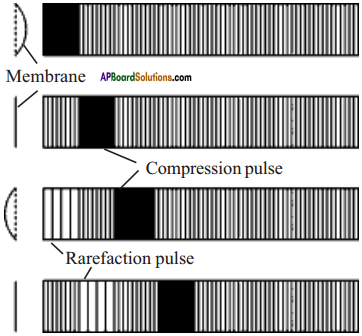• So, the particle of air in the layer get closer to each other, hence the density increases.
• This layer of air pushes and compresses the layer next to it and so on.
• We call this disturbance as compression pulse.
• When the membrane moves backward, it drags back the layer of air near it. Hence the density decreases.
• The particles of air in the next layer on the right move into fill this less dense area.
• This is a rarefaction pulse moves to right.
• As the membrane moves back and forth repeatedly, compression and rarefaction pulses are produced, one after the other.
• These two pulses travel one behind the other, carrying the disturbance with it.

Question 20.
How do echoes in a normal room affect the quality of the sounds that we hear? (AS 7)

• Echo is a reflected sound, arriving at the position of listener more than 0.1s after the direct sound.
• Quality is the characteristic of a sound which enables us to distinguish between musical notes emitted by different musical instruments.
• In a normal room, if echo is formed, we can hear multiple sounds, at same time.
• Our ear cannot perceive and judge the sound from where it is coming.
• So, quality of sound does not work here.

Question 21.
Explain the working and applications of SONAR. (AS 1)

• SONAR stands for Sonographic Navigation And Ranging.
• This is a method for detecting and finding the distance of objects under water by means of reflected ultrasonic waves.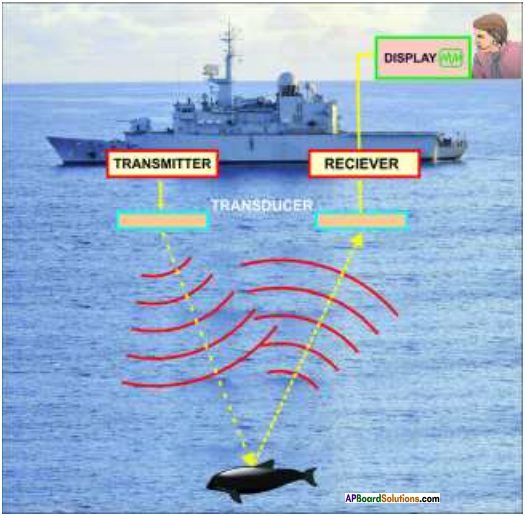Working of SONAR:

1. SONAR system consists of a transmitter and a detector in the “Observation Centre” on board of a ship.
2. From the observation centre, ultrasonic waves of high frequency are sent in all directions under the water through transmitter.
3. These waves travel in straight lines till they hit an object such as a submarine, a sunken ship, etc.
4. The waves are then reflected and are received back by the receiver at the observation centre.
5. The study of these reflected waves gives information about the direction of the object located.
6. The time between sending ultrasonic wave and receiving its echo, the distance of the object is calculated.
7. Reflections from various angles can be utilized to determine the shape and size of the object.

Mathematical expression :

1. Let’d’ be the distance between SONAR and an underwater object.
2. ‘t’ be the time between sending an ultrasonic wave and receiving its echo.
3. ‘u’ be the speed of sound in water.
4. The total distance covered by the wave from the SONAR to the object and back is 2d.
5. From the equation s = ut ⇒ 2d = ut ⇒ d = $$\frac{ut}{2}$$.

Application:
Marine geologists use this method to determine the depth of the sea and to locate underwater hills and valleys.Question 22.
Find the time period of a source of a sound wave whose frequency is 400 Hz. (AS 1)
Frequency υ = 400 Hz
Time period T =?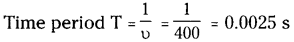Question 23.
A sound wave travels at a speed of 340 m/s. If its wavelength is 2 cm, what is the frequency of the wave? Will it be in the audible range? (AS 1)
Speed of sound v = 340 m/s.; Wavelength λ = 2 cm = 0.02 m.
Frequency υ =?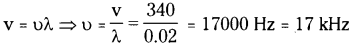The audible range of sound wave is 20 Hz to 20 kHz.
Hence this is in the audible range.

Question 24.
Given that sound travels in air at 340 m/s, find the wavelength of the waves in air produced by a 20 kHz sound source. If the same source is put in a water tank, what would be the wavelength of the sound waves in water? (AS 7)
Speed of sound in water = 1,480 m/s.
In air:
Speed of sound wave (v) = 340 m/s ; Frequency of source of sound (o) = 20 kHz
Wavelength of the sound wave λ =?
v = υλ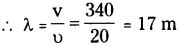∴ Wavelength of the sound wave in air = 17 m

Same source is kept in water :
∴ Speed of sound in water (v) = 1480 m/s
Frequency of sound wave (p) = 20 kHz
Wavelength of sound wave λ =?
v = υλ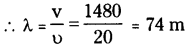∴ Wavelength of the sound wave in water = 74 mQuestion 25.
A man is lying on the floor of a large, empty hemispherical hall, in such a way that his head is at the centre of the hall. He shouts “Hello!” and hears the echo of his voice after 0.2 s. What is the radius of the hall ? (Speed of sound in air 340 m/s) (AS 7)
Let the distance travelled by the sound wave = 2d m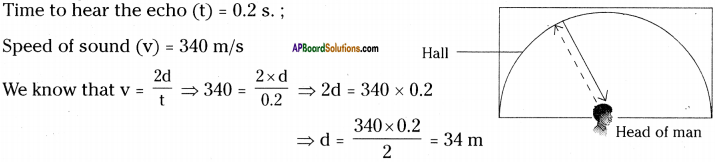As the head of the man is at the centre of hemispherical room, then ‘d’ is the radius of the hall.
∴ Radius of the hall = 34 m

Question 26.
“We know that sound is a form of energy. So, the large amount of energy produced due the sound pollution in cosmopolitan cities can be used to our day-to-day needs of energy. It also helps us to protect biodiversity in urban areas”. Do you agree with this statement? Explain.

1. Sound is a form of mechanical energy.
2. So, the mechanical energy can be converted into electrical energy.
3. Experiments are going on this concept.
4. If this is successful, we have the following benefits.
a) Sound pollution can be controlled.
b) Conventional methods of producing electrical energy from coal or water will cause in loss of biodiversity. This can be avoided.
c) Natural resources like water can be protected.
d) Increasing needs of energy can be overcome by this method.

Question 27.
How do you appreciate efforts of a musician to produce melodious sound using a musical instrument by simultaneously controlling frequency and amplitude of the sounds produced by it.

• The sounds which produce pleasing effect on the ear are called musical sounds.
• Any instrument which produces musical sound is called musical instrument.
• The person who plays a musical instrument to produce melodious sound is called a musician.
• The musician must have control on breathing, concentration on the output of the sound, which is a very hard task.
• For this the musician needs a lot of practise.
• With the musician’s practise and knowledge over musical notes only we can hear melodious sound otherwise it could only be a noise.
• Hence the efforts of a musician are highly appreciable.Question 28.
You might have observed that sometimes your pet dog starts barking though no one is seen near in its surroundings or no disturbance heard nearby. Does this observation raise any doubts in your mind about the peculiar behaviour of dog after your understanding about ‘range of hearing the sound’. If yes, write them.

• Dogs can hear sounds of frequencies up to about 50 kHz, which is ultrasonic.
• After hearing this ultrasonics, a dog will bark panicly, though no one is seen near.
• I understood this after studying about ‘range of hearing the sound’.
• Before the knowledge of ‘range of hearing the sound’, I felt that the dogs are barking by seeing some devils, which is a misconception.
• Scientific knowledge helps us to know reasons for many misconcepts.

Question 29.
Find out the names of animals (and their photographs from internet) which communicate using infra-sonic or ultra-sonic sound and prepare a scrap book.
Scrap book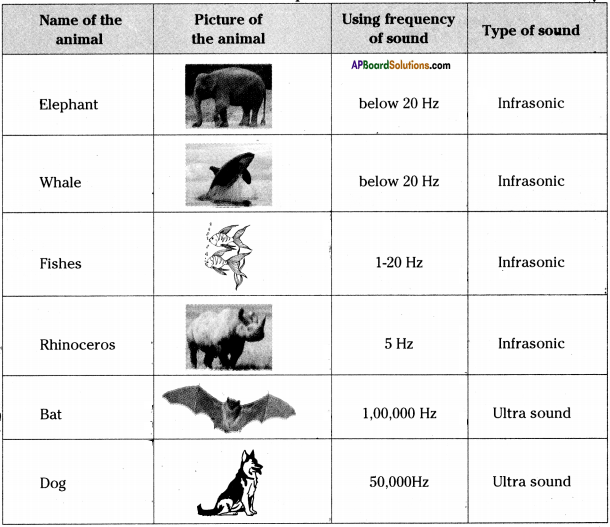### 9th Class Physical Science 11th Lesson Sound InText Questions and Answers

9th Class Physical Science Textbook Page No. 184

Question 1.
How does sound reach our ears from the source of its production?
Soufid travels in the form of waves. It reaches our ears from the source of its production, in the form of waves.

Question 2.
Does it travel by itself or is there any force bringing it to our ears?
Sound does not travel by itself. When a sound is produced, the kinetic energy of the source, vibrates the nearest particles in the medium. These particles transfers energy and finally, it reaches our ears.Question 3.
What is sound? Is it a force or an energy?
Sound is a form of energy.

Question 4.
Why don’t we hear sounds when our ears are closed?
When our ears are closed, the energy in the form of waves reaches our ear, but it cannot make the eardrum to vibrate. Hence we cannot hear the sound.

Question 5.
Why is the light ray dancing, after sound is made in the tin?
The dancing of light ray, after the sound is made in the tin shows that the sound travels in the form of waves from the source of its production.

Question 6.
What do you infer from this?
Sound is a form of energy which can travel in the form of waves through the medium.

Question 7.
Can we say that sound is a form of mechanical energy?
Yes, sound is a form of mechanical energy.

9th Class Physical Science Textbook Page No. 185

Question 8.
Do you hear any sound?
We cannot hear any sound.

Question 9.
Do you see any vibrations in the tuning fork?
Yes, we can sense the vibrations in the tuning fork.Question 10.
What do you conclude from the above activity?
We observe that vibrating tuning fork produces sound.

Question 11.
Can you produce sound without vibration in the body?
We cannot produce sound without vibration in the body.

Question 12.
Give some examples of vibrating bodies which produce sound.
Drums, tabla, calling bell, school bell, etc.

Question 13.
What part of our body vibrates when we speak?
When we speak, vocal cord vibrates in our body.Question 14.
Do all vibrating bodies necessarily produce sound?
All vibrating bodies produce sound, but we cannot hear some of them, due to the limit of audible frequency.

9th Class Physical Science Textbook Page No. 186

Question 15.
If sound travels in the form of a wave then what is the pattern?
Sound travels in the form of longitudinal or transverse waves in the air or in the other material.

9th Class Physical Science Textbook Page No. 188

Question 16.
What do you say about sound waves in air by the above activity?
From the above activity, we can say that there involves change in the density of medium while sound waves are travelling in air.

Question 17.
Are they longitudinal or transverse?
Sound waves in air are longitudinal.Question 18.
Does sound get reflected at the surface of a solid?
Yes, sound gets reflected at the surface of a solid as in the case of reflection of light.

Question 19.
What happens if you lift your tube slightly above the table?
If we lift the tube slightly we cannot hear the sound clearly.

Question 20.
Are able to listen to the sound? If not why?
We are unable to hear the sound. If we lift one of the pipes then the pipe carrying incident sound, the pipe carrying reflected sound will not be in the same plane. Hence we cannot hear the sound.

9th Class Physical Science Textbook Page No. 195

Question 21.
Do hard surfaces reflect sound better than soft ones?
Generally, hard surfaces reflect sound better than soft surfaces. But sound reflects quite well from rough surfaces than polished surfaces.

9th Class Physical Science Textbook Page No. 187

Question 22.
Do compressions and rarefactions in sound wave travel in same directions or in opposite directions? Explain.

• Compressions and rarefactions in a sound wave will be in opposite direction.
• In a compression, all the particles come close, so the density and pressure increases.
• In a rarefaction, all particles drag back, so the density and pressure decreases.
• In a microscopic view of particle, the compression and rarefaction travel in opposite directions.

9th Class Physical Science Textbook Page No. 191

Question 23.
Does the frequency of sound waves depend on the medium in which it frawels? How?
Yes.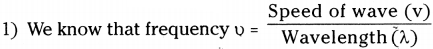2) As speed of wave differs from medium to fnedium, the frequency also changes, keeping the wavelengths constant.

Question 24.
The frequency of source of sound is 10 Hz. How many times does it vibrate in one minute?
Number of vibrations per second = 10
Number of vibrations in one minute = 10 × 60 = 600Question 25.
Gently strike a hanging bell (temple bell) and try to listen to the sound produced by it with a stethoscope keeping it both at bottom portion and top portion of the bell. Is the pitch and loudness of the sound same at the two portions? Why?
No. The bob of the bell strikes at the bottom portion of the bell. Hence the pitch and loudness are high.

Question 26.
During a thunderstorm if you note a 3 second delay between the flash of lightning and sound of thunder. What is the approximate distance of thunderstorm from you ?
Time taken to reach the sound = 3 s ; Speed of sound in air = 343.2 m/sec.
Distance of thunderstorm = 343.2 x 3 = 1029.6 m

9th Class Physical Science Textbook Page No. 194

Question 27.
Two girls are playing on identical stringed instruments. The strings of the both instruments are adjusted to give notes of same pitch. Will the quality of two notes be same? Justify your answer.
If the two girls are playing with same instruments, then the quality is same. If the girls are playing with different stringed instruments the quality will be different.

Quality is the characteristic which enables us to distinguish between musical notes emitted by different musical instruments.

Question 28.
What change, would you expect in the characteristic of a musical sound when we increase its frequency one instance and amplitude in another instance?
When frequency is increased, the pitch of sound increases. When amplitude is increased, its loudness increases.

9th Class Physical Science Textbook Page No. 195

Question 29.
What could be the reason for better reflection of sound by rough surfaces than polished surfaces?

• Sound reflects better on rough surfaces than polished surfaces.
• The rough surface reflects sound in all directions, so it can reach in many directions.

9th Class Physical Science Textbook Page No. 196

Question 30.
Why is an echo weaker than the original sound?

• Echo is the reflected sound.
• While it travels back, it gradually loses its energy.
• Hence the echo is weaker than original sound.Question 31.
In a closed box if you say hello, the sound heard will be Hellooooo ………. What does it mean?
In a closed box, the multiple reflections of sound has no way to go out side. Hence we will hear the sound as hellooooooo ………

9th Class Physical Science Textbook Page No. 197

Question 32.
What is the advantage of having conical openings in horns, megaphones, etc?
The conical openings in horns, megaphones, etc. will guide the reflected sound waves in forward direction and spreads towards the audience.

Question 33.
Why do we put cushions on the chairs, carpet on the floor, straw materials on the walls in cinema halls?

• These materials absorb unnecessary reflections of sound, so that we can hear more clearly.
• As these material absorb reflected sound waves, the people outside the cinema halls, cannot hear the sound produced in the cinema hall.

9th Class Physical Science Textbook Page No. 199

Question 34.
What is the benefit of using ultrasound over light waves in the above applications?

• Light waves cannot penetrate in the internal organs like liver, kidney, etc.
• Light wave after reflection do not form image.
• Ultrasound waves can penetrate through internal organs like liver, kidney, etc.
• After reflection, ultrasound waves produce image. So, in ultrasonography and surgeries, ultrasound waves are better than light waves.

9th Class Physical Science 11th Lesson Sound Activities

Activity 1

Question 1.
How can .you say that the sound is a form of energy?

1. Take a tin can and remove both ends to make a hollow cylinder.
2. Take a balloon and stretch it over the can.
3. Wrap a rubber band around the balloon.
4. Take a small mirror and stick it on the balloon.
5. Take a laser light and let it fall on the mirror.
6. After reflection the light spot is seen on the wall.
7. Now shout directly into the open end of the can and observe the dancing light.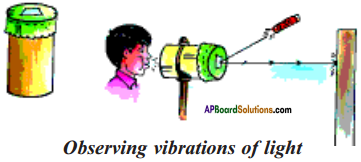Observations:

1. When sound is made, the energy produced from the sound vibrates the membrane of the balloon, resulting in the dancing of light ray.
2. This shows that sound is a form of energy which travels in air.

Activity – 2

Question 2.
Prove that the sound is produced from a vibrating source.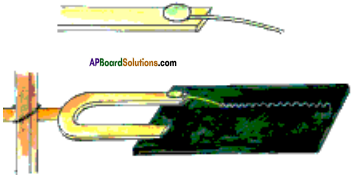1. Attach a small piece of steel wire to one of the prongs of a tuning fork as shown in the figure.
2. Strike the tuning fork with a rubber hammer.
3. While it is vibrating, try to draw a straight line on a piece of smoked glass as quick as possible with, it.
4. Keep the end of the wire in such a way just it touches the glass.
5. A line is formed in the form of a wave.
6. Repeat the experiment when the tuning fork is not vibrating and observe the difference in the line.

Observations:

1. We have produced vibrations in the tuning fork by striking it with a hammer.
2. Thus the vibrating tuning fork produces sound.
3. Thus the sound is produced by vibrating bodies.

Activity – 3

Question 3.
а) How do you demonstrate the formation of compressions and rarefactions in a slinky?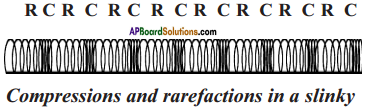2. It is a spring-shaped toy which can be extended or compressed very easily.
3. Lay it down on a table or the floor as shown in figure.
4. Ask a friend to hold one end.
5. Pull the other end to stretch the slinky and then move it to and fro along its length.
6. We will see alternate compressions and rarefactions of the coil.
7. This is similar to the pattern of varying density produced in a medium when sound passes through it.

b) Mow do you demonstrate the formation of crests and troughs in a slinky?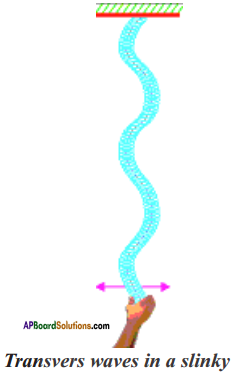1. Hang a slinky from a fixed support.
2. Hold it gently at the lower end and quickly move your hand sideways and back.
3. This will cause a hump on the slinky near the lower end.
4. The hump travels upwards on the slinky as shown in the figure.
5. The humps formed alternately are known as crests and troughs.

Activity – 4

Question 4.
Describe an activity to listen the reflected sound.1. Take two long, identical tubes and place them on
2. Ask your friend to speak softly into one tube while you use the other tube to listen.
3. Adjust the tube until you hear the best sound.
4. You will find that you hear your friend’s voice best when the tube makes equal angles with a normal to the wall.
5. This shows that reflection of sound follows the laws of reflection of light.
6. Lift your tube slightly above the table.
7. You will not be able to listen the voice clearly, because the plane carrying the incident wave and reflected wave are changed.
8. Repeat the experiment by placing flat objects of different materials (steel and plastic trays, a card board, a tray wrapped with cloth, etc.) against the wall and observe the changes in the sound.

Observations:

1. Reflection of sound follows the laws of reflection of light.
2. When the plane carrying incident wave and reflecting wave changes, the reflected sound cannot be heard clearly.
3. Hard or rough surfaces reflect the sound better than soft surfaces.